Next: Central schemes in one-space Up: A Short Guide to Previous: Upwind schemes

## Central schemes

As before, we seek a piecewise-polynomial,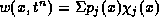, which serves as an approximate solution to the exact evolution of sliding averages in (1.2.4),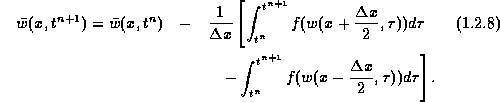Note that the polynomial pieces of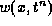are supported in the cells,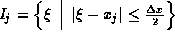, with interfacing breakpoints at the half-integers gridpoints,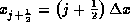.

We recall that upwind schemes (1.2.5) were based on sampling (1.2.4) in the midcells,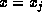. In contrast, central schemes are based on sampling (1.2.8) at the interfacing breakpoints,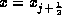, which yields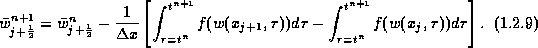We want to utilize (1.2.9) in terms of the known cell averages at time level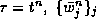. The remaining task is therefore to recover the pointvalues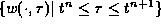, and in particular, the staggered averages,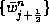. As before, this task is accomplished in two main steps:

• First, we use the given cell averages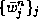, to reconstruct the pointvalues of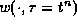as piecewise polynomial approximation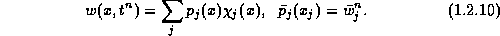In particular, the staggered averages on the right of (1.2.9) are given by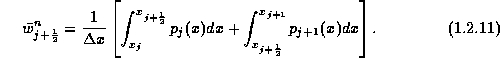The resulting central scheme (1.2.9) then reads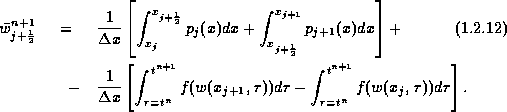• Second, we follow the evolution of the pointvalues along the mid-cells,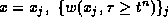, which are governed by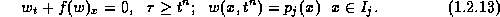Let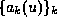denote the eigenvalues of the Jacobian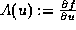. By hyperbolicity, information regarding the interfacing discontinuities at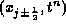propagates no faster than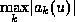. Hence, the mid-cells values governed by (1.2.13),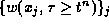, remain free of discontinuities, at least for sufficiently small time step dictated by the CFL condition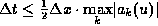. Consequently, since the numerical fluxes on the right of (1.2.12),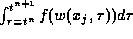, involve only smooth integrands, they can be computed within any degree of desired accuracy by an appropriate quadrature rule.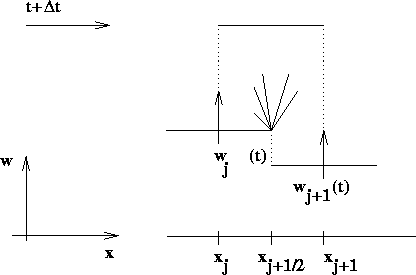Figure 1.2.2: Central differencing by Godunov-type scheme.

It is the staggered averaging over the fan of left-going and right-going waves centered at the half-integered interfaces,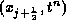, which characterizes the central differencing, consult Figure 1.2.2. A main feature of these central schemes - in contrast to upwind ones, is the computation of smooth numerical fluxes along the mid-cells,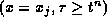, which avoids the costly (approximate) Riemann solvers. A couple of examples of central Godunov-type schemes is in order.

The first-order Lax-Friedrichs (LxF) approximation is the forerunner for such central schemes -- it is based on piecewise constant reconstruction,with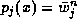. The resulting central scheme, (1.2.12), then reads (with the usual fixed mesh ratio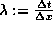)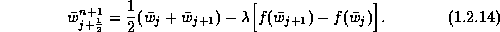Our main focus in the rest of this chapter is on non-oscillatory higher-order extensions of the LxF schemes.Next: Central schemes in one-space Up: A Short Guide to Previous: Upwind schemes